Request a Free Counselling Session from our Expert Mentor# NCERT Solution for Class Xth Science Chapter 10 Light, Reflection & Refraction

Welcome to the NCERT Solutions for Class X Science Chapter 10 – Light, Reflection & Refraction. This chapter is all about understanding the properties of light, how it interacts with different objects, and how it is reflected and refracted.
In this chapter, you will learn about the laws of reflection and refraction, the different types of mirrors and lenses, and the formation of images by them. You will also learn about the different phenomena of light like dispersion, scattering, and the blue color of the sky.
Our solutions are designed to help you understand the concepts thoroughly and prepare for your exams. We have provided step-by-step solutions to all the questions in the NCERT textbook, along with additional tips and tricks to help you score well in your exams.
At Swastik Classes, we believe in making learning fun and engaging. Our NCERT Solutions for Class X Science Chapter 10 – Light, Reflection & Refraction are designed to make the learning process enjoyable and easy for you. So, let’s get started and explore the fascinating world of light together!

## Answers of Science NCERT solutions for class 10 Chapter 10 Light, Reflection & Refraction

Chapter -10

Light, Reflection & Refraction

In-Chapter Exercise

Question 1:

Define the principal focus of a concave mirror.

Light rays that are parallel to the principal axis of a concave mirror converge at a specific point on its principal axis after reflecting from the mirror. This point is known as the principal focus of the concave mirror.

Question 2:

The radius of curvature of a spherical mirror is 20 cm. What is its focal length? Answer:

Radius of curvature, R = 20 cm

Radius of curvature of a spherical mirror = 2 × Focal length (f)

R = 2f

Hence, the focal length of the given spherical mirror is 10 cm.

Question 3:

Name the mirror that can give an erect and enlarged image of an object.

When an object is placed between the pole and the principal focus of a concave mirror, the image formed is virtual, erect, and enlarged.

*Question 4:

Why do we prefer a convex mirror as a rear-view mirror in vehicles?

Convex mirrors give a virtual, erect, and diminished image of the objects placed in front of them. They are preferred as a rear-view mirror in vehicles because they give a wider field of view, which allows the driver to see most of the traffic behind him.

In-Chapter Exercise

Question 1:

Find the focal length of a convex mirror whose radius of curvature is 32 cm.

Radius of curvature, R = 32 cm

Radius of curvature = 2 × Focal length (f)

R = 2f

Hence, the focal length of the given convex mirror is 16 cm.

*Question 2:

A concave mirror produces a three times magnified (enlarged) real image of an object placed at 10 cm in front of it. Where is the image located?

Magnification produced by a spherical mirror is given by the relation,

Let the height of the object, ho = h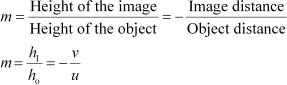Then, height of the image, hI = −3h (Image formed is real)

Object distance, u = −10 cm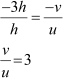v = 3 × (−10) = −30 cm

Here, the negative sign indicates that an inverted image is formed at a distance of 30 cm in front of the given concave mirror.

In-Chapter Exercise

*Question 1:

A ray of light travelling in air enters obliquely into water. Does the light ray bend towards the normal or away from the normal? Why?

The light ray bends towards the normal.

When a ray of light travels from an optically rarer medium to an optically denser medium, it gets bent towards the normal. Since water is optically denser than air, a ray of light travelling from air into the water will bend towards the normal.

*Question 2:

Light enters from air to glass having refractive index 1.50. What is the speed of light in the glass? The speed of light in vacuum is 3 × 108 m s−1.

Refractive index of a medium nm is given by,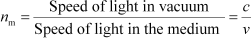Speed of light in vacuum, c = 3 × 108 m s−1 Refractive index of glass, ng = 1.50

Speed of light in the glass,

Question 3:

Find out, from Table, the medium having highest optical density. Also find the medium with lowest optical density.

Highest optical density =

Diamond Lowest optical density = Air

Optical density of a medium is directly related with the refractive index of that medium. A medium which has the highest refractive index will have the highest optical density and vice-versa.

It can be observed from table 10.3 that diamond and air respectively have the highest and lowest refractive index. Therefore, diamond has the highest optical density and air has the lowest optical density.

Question 4:

You are given kerosene, turpentine and water. In which of these does the light travel fastest? Use the information given in Table.

Speed of light in a medium is given by the relation for refractive index (nm). The relation is given as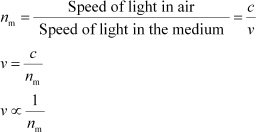It can be inferred from the relation that light will travel the slowest in the material which has the highest refractive index and travel the fastest in the material which has the lowest refractive index.

It can be observed from table 10.3 that the refractive indices of kerosene, turpentine, and water are 1.44, 1.47, and 1.33 respectively. Therefore, light travels the fastest in water.

*Question 5:

The refractive index of diamond is 2.42. What is the meaning of this statement?

Refractive index of a medium nm is related to the speed of light in that medium v by the relation.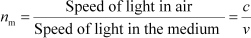Where, c is the speed of light in vacuum/air

The refractive index of diamond is 2.42. This suggests that the speed of light in diamond will reduce by a factor 2.42 compared to its speed in air.

In-Chapter Exercise

*Question 1:

Define 1 dioptre of power of a lens.

Power of lens is defined as the reciprocal of its focal length. If P is the power of a lens of focal length F in metres, then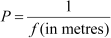The S.I. unit of power of a lens is Dioptre. It is denoted by D.

1 dioptre is defined as the power of a lens of focal length 1 metre.

∴1 D = 1 m−1

*Question 2:

A convex lens forms a real and inverted image of a needle at a distance of 50 cm from it. Where is the needle placed in front of the convex lens if the image is equal to the size of the object? Also, find the power of the lens.

When an object is placed at the centre of curvature, 2F1, of a convex lens, its image is formed at the centre of curvature, 2F2, on the other side of the lens. The image formed is inverted and of the same size as the object, as shown in the given figure.

It is given that the image of the needle is formed at a distance of 50 cm from the convex lens. Hence, the needle is placed in front of the lens at a distance of

50 cm.

Object distance, u = −50 cm Image distance, v = 50 cm Focal length = f

According to the lens formula,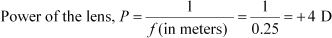Hence, the power of the given lens is +4 D.

Question 3:

Find the power of a concave lens of focal length 2 m.

Focal length of concave lens, f = 2 m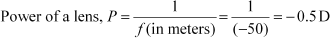Here, negative sign arises due to the divergent nature of concave lens. Hence, the power of the given concave lens is −0.5 D.

Last Exercise

Question 1:

Which one of the following materials cannot be used to make a lens?

1. Water
2. Glass
3. Plastic
4. Clay

(d) A lens allows light to pass through it. Since clay does not show such property, it cannot be used to make a lens.

*Question 2:

The image formed by a concave mirror is observed to be virtual, erect and larger than the object. Where should be the position of the object?

1. Between the principal focus and the centre of curvature
2. At the centre of curvature
3. Beyond the centre of curvature
4. Between the pole of the mirror and its principal focus.

(d) When an object is placed between the pole and principal focus of a concave mirror, the image formed is virtual, erect, and larger than the object.

Question 3:

Where should an object be placed in front of a convex lens to get a real image of the size of the object?

1. At the principal focus of the lens
2. At twice the focal length
3. At infinity
4. Between the optical centre of the lens and its principal focus.

(b) When an object is placed at the centre of curvature in front of a convex lens, its image is formed at the centre of curvature on the other side of the lens. The image formed is real, inverted, and of the same size as the object.

*Question 4:

A spherical mirror and a thin spherical lens have each a focal length of −15 cm. The mirror and the lens are likely to be

1. both concave
2. both convex
3. the mirror is concave and the lens is convex
4. the mirror is convex, but the lens is concave

(a) By convention, the focal length of a concave mirror and a concave lens are taken as negative. Hence, both the spherical mirror and the thin spherical lens are concave in nature.

Question 5:

No matter how far you stand from a mirror, your image appears erect. The mirror is likely to be

1. plane
2. concave
3. convex
4. either plane or convex

(d) A convex mirror always gives a virtual and erect image of smaller size of the object placed in front of it. Similarly, a plane mirror will always give a virtual and erect image of same size as that of the object placed in front of it. Therefore, the given mirror could be either plane or convex.

*Question 6:

Which of the following lenses would you prefer to use while reading small letters found in a dictionary?

1. A convex lens of focal length 50 cm
2. A concave lens of focal length 50 cm
3. A convex lens of focal length 5 cm
4. A concave lens of focal length 5 cm

(c) A convex lens gives a magnified image of an object when it is placed between the

radius of curvature and focal length. Also, magnification is more for convex lenses having shorter focal length. Therefore, for reading small letters, a convex lens of focal length 5 cm should be used.

Question 7:

We wish to obtain an erect image of an object, using a concave mirror of focal length 15 cm. What should be the range of distance of the object from the mirror? What is the nature of the image? Is the image larger or smaller than the object? Draw a ray diagram to show the image formation in this case.

Range of object distance = 0 cm to15 cm

A concave mirror gives an erect image when an object is placed between its pole (P) and the principal focus (F).

Hence, to obtain an erect image of an object from a concave mirror of focal length 15 cm, the object must be placed anywhere between the pole and the focus. The image formed will be virtual, erect, and magnified in nature, as shown in the given figure.

*Question 8:

Name the type of mirror used in the following situations.

2. Side/rear-view mirror of a vehicle
3. Solar furnace

(a) Concave (b) Convex (c) Concave

Explanation

1. Concave mirror is used in the headlights of a car. This is because concave mirrors can produce powerful parallel beam of light when the light source is placed at their principal focus.
2. Convex mirror is used in side/rear view mirror of a vehicle. Convex mirrors give a virtual, erect, and diminished image of the objects placed in front of it. Because of this, they have a wide field of view. It enables the driver to see most of the traffic behind him/her.
3. Concave mirrors are converging mirrors. That is why they are used to construct solar furnaces. Concave mirrors converge the light incident on them at a single point known as principal focus. Hence, they can be used to produce a large amount of heat at that point.

*Question 9:

One-half of a convex lens is covered with a black paper. Will this lens produce a complete image of the object? Verify your answer experimentally. Explain your observations.

The convex lens will form a complete image of an object, even if it’s one half is covered with black paper. It can be understood by the following two cases.

Case I

When the upper half of the lens is covered

In this case, a ray of light coming from the object will be refracted by the lower half of the lens. These rays meet at the other side of the lens to form the image of the given object, as shown in the following figure.

Case II

When the lower half of the lens is covered

In this case, a ray of light coming from the object is refracted by the upper half of the lens. These rays meet at the other side of the lens to form the image of the given object, as shown in the following figure.

*Question 10:

An object 5 cm in length is held 25 cm away from a converging lens of focal length 10 cm. Draw the ray diagram and find the position, size and the nature of the image formed.

Object distance, u = −25 cm Object height, ho = 5 cm Focal length, f = +10 cm According to the lens formula,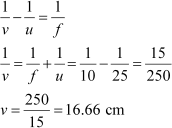The positive value of v shows that the image is formed at the other side of the lens.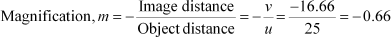The negative sign shows that the image is real and formed behind the lens.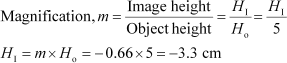The negative value of image height indicates that the image formed is inverted. The position, size, and nature of image are shown in the following ray diagram.

Question 11:

A concave lens of focal length 15 cm forms an image 10 cm from the lens. How far is the object placed from the lens? Draw the ray diagram.

Focal length of concave lens (OF1), f = −15 cm Image distance, v = −10 cm

According to the lens formula,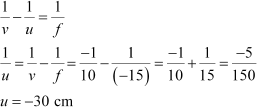The negative value of u indicates that the object is placed 30 cm in front of the lens. This is shown in the following ray diagram.

Question 12:

An object is placed at a distance of 10 cm from a convex mirror of focal length 15 cm. Find the position and nature of the image.

Focal length of convex mirror, f = +15 cm

Object distance, u = −10 cm

According to the mirror formula,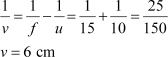The positive value of v indicates that the image is formed behind the mirror.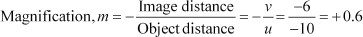The positive value of magnification indicates that the image formed is virtual and erect.

Question 13:

The magnification produced by a plane mirror is +1. What does this mean?

Magnification produced by a mirror is given by the relation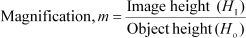The magnification produced by a plane mirror is +1. It shows that the image formed by the plane mirror is of the same size as that of the object. The positive sign shows that the image formed is virtual and erect.

Question 14:

An object 5.0 cm in length is placed at a distance of 20 cm in front of a convex mirror of radius of curvature 30 cm. Find the position of the image, its nature and size.

Object distance, u = −20 cm Object height, h = 5 cm

Radius of curvature, R = 30 cm

Radius of curvature = 2 × Focal length

R = 2f

f = 15 cm

According to the mirror formula,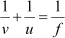The positive value of v indicates that the image is formed behind the mirror.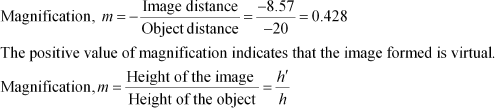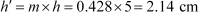The positive value of image height indicates that the image formed is erect. Therefore, the image formed is virtual, erect, and smaller in size.

*Question 15:

An object of size 7.0 cm is placed at 27 cm in front of a concave mirror of focal length 18 cm. At what distance from the mirror should a screen be placed, so that a sharp focused image can be obtained? Find the size and the nature of the image.

Object distance, u = −27 cm Object height, h = 7 cm Focal length, f = −18 cm

According to the mirror formula,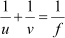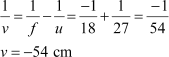The screen should be placed at a distance of 54 cm in front of the given mirror.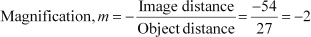The negative value of magnification indicates that the image formed is real.

The negative value of image height indicates that the image formed is inverted.

*Question 16:

Find the focal length of a lens of power −2.0 D. What type of lens is this?

Answer: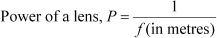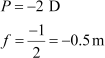A concave lens has a negative focal length. Hence, it is a concave lens.

*Question 17:

A doctor has prescribed a corrective lens of power +1.5 D. Find the focal length of the lens. Is the prescribed lens diverging or converging?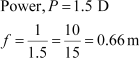A convex lens has a positive focal length. Hence, it is a convex lens or a converging lens.

### Access Chapter wise NCERT Solutions for Class 10 Science Light Reflection and Refraction (All In-text and Exercise Questions Solved)

Questions Page: 168

1. Define the principal focus of a concave mirror.

Light rays that are parallel to the principal axis of a concave mirror converge at a specific point on its principal axis after reflecting from the mirror. This point is called the principal focus of the concave mirror.

2. The radius of curvature of a spherical mirror is 20 cm. What is its focal length?

Radius of curvature (R) = 20 cm

Radius of curvature of the spherical mirror = 2 × Focal length (f)

R = 2f

f= R/2 = 20 / 2 = 10

Therefore, the focal length of the spherical mirror is 10 cm.

3. Name the mirror that can give an erect and enlarged image of an object.

The mirror that can give an erect and enlarged image of an object is Concave Mirror.

4. Why do we prefer a convex mirror as a rear-view mirror in vehicles?

Convex mirror is preferred as a rear-view mirror in cars and vehicles as it gives a wider field of view, which helps the driver to see most of the traffic behind him. Convex mirrors always form an erect, virtual, and diminished image of the objects placed in front of it.

Page No: 171

1. Find the focal length of a convex mirror whose radius of curvature is 32 cm.

Radius of curvature (R) = 32 cm

Radius of curvature = 2 × Focal length (f)

R= 2f

f = R/2 = 32/2 = 16

Therefore, the focal length of the given convex mirror is 16 cm.

2. A concave mirror produces three times magnified (enlarged) real image of an object placed at 10 cm in front of it. Where is the image located?

Magnification produced by a spherical mirror:

Object distance (u) = – 10 cm

v = 3 × (- 10) = – 30 cm

Therefore, the negative sign indicates that an inverted image is formed in front of the given concave mirror at a distance of 30 cm.

Page No: 176

1. A ray of light travelling in air enters obliquely into water. Does the light ray bends towards the normal or away from the normal? Why?

The light ray bends towards the normal. When a light ray enters from an optically rarer medium (which has low refractive index) to an optically denser medium (which has a high refractive index), its speed slows down and bends towards the normal. As water is optically denser than air, a ray of light entering from air into water will bend towards the normal.

2. Light enters from air to glass having refractive index 1.50. What is the speed of light in the glass? The speed of light in vacuum is 3 x 108 ms-1.

Refractive index of a medium (nm) = Speed of light in vacuum/Speed of light in the medium

Speed of light in vacuum (c) = 3 × 108 m/s

Refractive index of glass (ng) = 1.50

Speed of light in the glass (v) = Speed of light in vacuum/ Refractive index of glass

= c/ng

=3 × 108/1.50 = 2x 108 ms-1.

3. Find out, from Table, the medium having highest optical density. Also find the medium with lowest optical density.

Lowest optical density = Air

Highest optical density = Diamond

The optical density of a medium is directly related to its refractive index. A medium with the highest refractive index will have the highest optical density and vice-versa.

It can be observed from the table that air and diamond respectively have the lowest and highest refractive index. Hence, air has the lowest optical density and diamond has the highest optical density.

4. You are given kerosene, turpentine and water. In which of these does the light travel fastest? Use the information given in Table.

Light travel faster in water as compared to kerosene & turpentine as the refractive index of water is lower than that of kerosene and turpentine. The speed of light is inversely proportional to the refractive index.

5. The refractive index of diamond is 2.42. What is the meaning of this statement?

Diamond has a refractive index of 2.42 which means that the speed of light in diamond will reduce by a factor of 2.42 as compared to its speed in the air.

In other words, the speed of light in diamond is 1/2.42 times the speed of light in vacuum.

Page No: 184

1. Define 1 dioptre of power of a lens.

Dioptre is the SI unit of power of lens is denoted by the letter D. 1 dioptre can be defined as the power of a lens of focal length 1 metre.

2. A convex lens forms a real and inverted image of a needle at a distance of 50 cm from it. Where is the needle placed in front of the convex lens if the image is equal to the size of the object? Also, find the power of the lens.

The position of the image should be at 2F since the image is the real and same size.

It is given that the image of the needle is formed at a distance of 50 cm from the convex lens. Therefore, the needle is placed in front of the lens at a distance of 50 cm.

Object distance (u) = – 50 cm

Image distance, (v) = 50 cm

Focal length = f

According to the lens formula,

3. Find the power of a concave lens of focal length 2 m.

Focal length of concave lens (f) = 2 m

Power of lens (P) = 1/f = 1/ (-2) = -0.5D

Page No: 185

Exercise

1. Which one of the following materials cannot be used to make a lens?

(a) Water

(b) Glass

(c) Plastic

(d) Clay

(d) Clay cannot be used to make a lens because if the lens is made up of clay the light rays cannot pass through it

2. The image formed by a concave mirror is observed to be virtual, erect and larger than the object. Where should be the position of the object?

(a) Between the principal focus and the centre of curvature

(b) At the centre of curvature

(c) Beyond the centre of curvature

(d) Between the pole of the mirror and its principal focus.

(d) The position of the object should be between the pole of the mirror and its principal focus.

3. Where should an object be placed in front of a convex lens to get a real image of the size of the object?

(a) At the principal focus of the lens

(b) At twice the focal length

(c) At infinity

(d) Between the optical centre of the lens and its principal focus.

(b) The object should be placed at twice the focal length

4. A spherical mirror and a thin spherical lens have a focal length of -15 cm. The mirror and the lens are likely to be

(a) both concave

(b) both convex

(c) the mirror is concave and the lens is convex

(d) the mirror is convex, but the lens is concave

(a) Both are likely to be concave.

Page No: 186

5. No matter how far you stand from a mirror, your image appears erect. The mirror is likely to be

(a) plane

(b) concave

(c) convex

(d) either plane or convex

(d) The mirrors are likely to be either plane or convex

6. Which of the following lenses would you prefer to use while reading small letters found in a dictionary?

(a) A convex lens of focal length 50 cm

(b) A concave lens of focal length 50 cm

(c) A convex lens of focal length 5 cm

(d) A concave lens of focal length 5 cm

(c) A convex lens of focal length 5 cm can be used while reading small letters found in a dictionary

7. We wish to obtain an erect image of an object, using a concave mirror of focal length 15 cm. What should be the range of distance of the object from the mirror? What is the nature of the image? Is the image larger or smaller than the object? Draw a ray diagram to show the image formation in this case.

Range of the distance of the object = 0 to 15 cm from the pole of the mirror.

Nature of the image = virtual, erect, and larger than the object.

8. Name the type of mirror used in the following situations.

(b) Side/rear-view mirror of a vehicle

(c) Solar furnace

(a) Concave Mirror: Because concave mirrors can produce a powerful parallel beam of light when the light source is placed at their principal focus.

(b) Convex Mirror: Because of its largest field of view.

(c) Concave Mirror: Because it concentrates the parallel rays of the sun at a principal focus.

9. One-half of a convex lens is covered with a black paper. Will this lens produce a complete image of the object? Verify your answer experimentally. Explain your observations.

Yes, it will produce a complete image of the object, as shown in the figure. This can be verified experimentally by observing the image of a distant object like a tree on a screen when the lower half of the lens is covered with a black paper. However, the intensity or brightness of the image will reduce.

10. An object 5 cm in length is held 25 cm away from a converging lens of focal length 10 cm. Draw the ray diagram and find the position, size and the nature of the image formed.

Height of the Object, h0 = 5 cm

Distance of the object from converging lens, u = -25 cm

Focal length of a converging lens, f = 10 cm

Using lens formula,

Thus, the image is inverted and formed at a distance of 16.7 cm behind the lens and measures 3.3 cm. The ray diagram is shown below.

11. A concave lens of focal length 15 cm forms an image 10 cm from the lens. How far is the object placed from the lens? Draw the ray diagram.

Focal length of concave lens (OF1), f = – 15 cm

Image distance, v= – 10 cm

According to the lens formula,

The negative value of u indicates that the object is placed 30 cm in front of the lens. This is shown in the following ray diagram.

12. An object is placed at a distance of 10 cm from a convex mirror of focal length 15 cm. Find the position and nature of the image.

Focal length of convex mirror (f) = +15 cm

Object distance (u) = – 10 cm

According to the mirror formula,

The image is located at a distance of 6 cm from the mirror on the other side of the mirror.

The positive and a value of less than 1 of magnification indicates that the image formed is virtual and erect and diminished.

13. The magnification produced by a plane mirror is +1. What does this mean?

The positive sign means an image formed by a plane mirror is virtual and erect. Since the magnification is 1 it means that the size of the image is equal to the size of the object.

14. An object 5 cm is placed at a distance of 20 cm in front of a convex mirror of radius of curvature 30 cm. Find the position, nature and size of the image.

Object distance (u) = – 20 cm

Object height (h) = 5 cm

Radius of curvature (R) = 30 cm

Radius of curvature = 2 × Focal length

R = 2f

f = 15 cm

According to the mirror formula,

The positive value of image height indicates that the image formed is erect.

Hence, the image formed is erect, virtual, and smaller in size.

15. An object of size 7.0 cm is placed at 27 cm in front of a concave mirror of focal length 18 cm. At what distance from the mirror should a screen be placed so that a sharply focused image can be obtained? Find the size and nature of the image.

Object distance (u) = – 27 cm

Object height (h) = 7 cm

Focal length (f) = – 18 cm

According to the mirror formula,

The negative value of image height indicates that the image formed is inverted.

16. Find the focal length of a lens of power -2.0 D. What type of lens is this?

Power of lens (P) = 1/f

P = -2D

f = -1/2 = -0.5 m

A concave lens has a negative focal length. Therefore, it is a concave lens.

17. A doctor has prescribed a corrective lens of power +1.5 D. Find the focal length of the lens. Is the prescribed lens diverging or converging?

Power of lens (P) = 1/f

P = 1.5D

f = 1/1.5 = 10/15 = 0.66 m

A convex lens has a positive focal length. Therefore, it is a convex lens or a converging lens.

## NCERT Solutions For Class 10 Science Chapter 10 Light Reflection and Refraction

Chapter 10 Light Reflection and Refraction are one of the important chapters in Class 10 Science and the expected marks weightage of the chapter according to the latest marking scheme is 7 marks. In Chapter 10 of Class 10 Science, students will get well versed with light phenomena such as refraction and reflection. One will learn about spherical mirrors, image formation and to draw ray diagrams.

Topics covered in this chapter:

• Reflection of Light
• Spherical Mirrors
• Image Formation by Spherical Mirrors
• Representation of Images Formed by Spherical Mirrors Using Ray Diagram – 4 Questions (2 short, 2 long)
• Mirror Formula and Magnification – 2 Questions (2 Numerical)
• Refraction of Light – 5 Questions (1 numerical, 2 Long answers and 1 Short answer)
• Power of Lens – 3 Questions (2 Short answers and 1 numerical)

Have you ever wondered what makes things visible to us? The answer to the question is light. During the day, it is sunlight that helps us see objects. When light falls on an object, it reflects. This reflected ray when received by our eyes helps us see things. There are numerous wonderful phenomena that are associated with light such as the formation of the rainbow, of stars, and many more. In Chapter 10 Light Reflection and Refraction of Class 10 Science, let us study the phenomena of refraction and reflection using straight-line propagation of light. We shall also try to understand the reflection of light by spherical mirrors in this chapter using the NCERT Solutions from SWC.

### Key Features of NCERT Solutions for Class 10 Science Chapter 10 Light Reflection and Refraction:

• Provides comprehensive answers to all the questions asked in the chapter
• The language used is lucid and can be understood by all
• The information provided is genuine and appropriate
• These solutions can be referred for CBSE exams, Olympiads and other competitive exams
• Concise answers provided to help students understand better

Conclusion

The NCERT Solutions for Class X Science Chapter 10 – Light, Reflection & Refraction provide a comprehensive understanding of the properties of light, how it interacts with different objects, and how it is reflected and refracted. The chapter emphasizes the importance of light and its various applications in our daily lives.
Our solutions provide step-by-step explanations to all the questions in the NCERT textbook, making it easy for students to understand the concepts. We have also provided additional tips and tricks to help students score well in their exams.
By studying this chapter, students will not only gain knowledge about the properties of light but also develop an understanding of their applications in fields like optics, astronomy, and photography. It is crucial for us to appreciate the beauty of light and understand the science behind it.
At Swastik Classes, we believe in providing a holistic learning experience to our students, and our NCERT Solutions for Class X Science Chapter 10 – Light, Reflection & Refraction are a testament to our commitment to quality education.

### Is the SWC NCERT Solutions for Class 10 Science Chapter 10 available in PDF format?

No, SWC provides the NCERT Solutions for Class 10 Science Chapter 10 in text format currently but in future we are planning to provide it in pdf format, which can be downloaded by the students. These reference materials from SWC are hassle-free and help students to score well in the Class 10 exams. The PDFs created by the experts are of high quality and the free download links can be used by the students to access them.